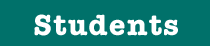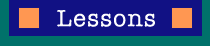Getting StartedBringing It HomeUnit Conversions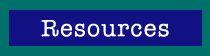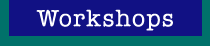Printer version

## Energized Learning: Unit Conversions

Team Number/Name: _______________ Date: __________

Student Name(s): _________________________________

Weather City: _______________ Zip Code: __________

1. Using the energy consumption values from the two "greenhouse gas emissions and energy consumption" tables (from Part I and Part II of the "Getting Started" lesson) and the two worksheets provided, calculate the total energy use of the house in common units (millions of BTUs/year) for the results in Parts I and Part II. Calculate total carbon dioxide (CO2) emissions from the house for the results in Parts I and II. Show how the units should be cancelled in the formulas.

2. Based on your results from the Worksheets in the previous question, do the carbon dioxide emissions found in Parts I and II decline from the results by the same percentage as the total energy use? If not, why would this be?

3. Do the emissions calculated on the worksheets agree with those on from the Home Energy Saver? (Yes/No). If not, why might this be?

### Energy Unit Conversions Worksheet: A

Complete this worksheet with the information found in Part I (base home), showing how the units cancel to yield the proper final units. Note: The home you are modeling will probably not have all four types of energy shown below! Just skip the ones that are not relevant.

 1. Convert raw energy to common units (millions of BTUs, MBTU): a. _______________ kWh x 3413 BTU/kWh x 1 MBTU/1,000,000 BTU = _______________ (fill in value and units) of electricity b. _______________ Therms x 100,000 BTU/therm x 1 MBTU/1,000,000 BTU = _______________ (fill in value and units) of natural gas c. _______________ Gallons Oil x 138,095 BTU/gallon x 1 MBTU/1,000,000 BTU = _______________ (fill in value and units) of heating oil d. _______________ Gallons LPG x 95,500 BTUs/gallon x 1 MBTU/1,000,000 BTU = _______________ (fill in value and units) of heating oil e. _______________ combine the preceding results to get total energy from all sources 2. Convert MBTU of energy to greenhouse-gas emissions (pounds of carbon dioxide, CO2) a. 1.2 lbs CO2/kWh x 1 kWh/0.003413 MBTU x __________ MBTU of electricity = _______________ (fill in value and units) CO2 from electricity b. 12 lbs CO2/therm x 1 therm/0.1 MBTU x __________ MBTU of natural gas = _______________ (fill in value and units) CO2 from natural gas c. 22.384 lbs CO2/gallon oil x 1 gallon/0.138095 MBTU x __________ MBTU of heating oil = _______________ (fill in value and units) CO2from heating oil d. 12.805 lbs CO2/gallon LPG x 1 gallon/0.0955 MBTU x __________ MBTU of LPG = _______________ (fill in value and units) CO2from LPG e. _______________ Combine the preceding results to get total CO2 from all sources of energy

### Energy Unit Conversions Worksheet: B

Complete this worksheet with the information found in Part II (efficient home). Show how the units cancel to yield the proper final results. Note: The home you are modeling will probably not have all four types of energy shown below! Just skip the ones that are not relevant.

 1. Convert raw energy to common units (millions of BTUs, MBTU): a. _______________ kWh x 3413 BTU/kWh x 1 MBTU/1,000,000 BTU = _______________ (fill in value and units) of electricity b. _______________ Therms x 100,000 BTU/therm x 1 MBTU/1,000,000 BTU = _______________ (fill in value and units) of natural gas c. _______________ Gallons Oil x 138,095 BTU/gallon x 1 MBTU/1,000,000 BTU = _______________ (fill in value and units) of heating oil d. _______________ Gallons LPG x 95,500 BTUs/gallon x 1 MBTU/1,000,000 BTU = _______________ (fill in value and units) of heating oil e. _______________ combine the preceding results to get total energy from all sources 2. Convert MBTU of energy to greenhouse-gas emissions (pounds of carbon dioxide, CO2) a. 1.2 lbs CO2/kWh x 1 kWh/0.003413 MBTU x __________ MBTU of electricity = _______________ (fill in value and units) CO2 from electricity b. 12 lbs CO2/therm x 1 therm/0.1 MBTU x __________ MBTU of natural gas = _______________ (fill in value and units) CO2 from natural gas c. 22.384 lbs CO2/gallon oil x 1 gallon/0.138095 MBTU x __________ MBTU of heating oil = _______________ (fill in value and units) CO2from heating oil d. 12.805 lbs CO2/gallon LPG x 1 gallon/0.0955 MBTU x __________ MBTU of LPG = _______________ (fill in value and units) CO2from LPG e. _______________ Combine the preceding results to get total CO2 from all sources of energy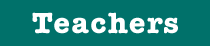Getting StartedBringing It HomeUnit Conversions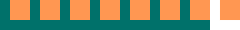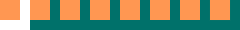Introduction | Students | Teachers | Credits Contact | Webmaster